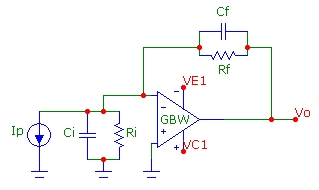Transimpedance Calculator
GBW (MHz):      Rf (k):    Ri (k):      Cf (pF):      Ci (pF):
f3db (Hz):   Q:   F0 (Hz):   Fz (Hz):   Fp (Hz):(see What's My Transimpedance Q and f3db Bandwidth?)

## Parameters

• GBW    op-amp gain-bandwidth product
• Rf    feedback resistance
• Ri    total shunt resistance at inverting-input to ground
• Cf    feedback shunt capacitance
• Ci    total capacitance at inverting-input to ground
• f3db    -3 dB bandwidth of transimpedance transfer function
• Q    quality factor of transimpedance transfer function
• F0    natural undamped resonant frequency of transimpedance transfer function
• Fz    zero frequency of noise-gain function
• Fp    pole frequency of noise-gain function